# Tటee5३/७334Groups24039. Let G b27. Let G be a finite group and let p be a prime. If p2> IGI, show thatany subgroup of order p is normal in G.28. Let G ZeZ and H = {(x, y) |x and y are eventhat H is a subgroup of G. Determine the order of G/H. To whichmeadintegers). ShowН-Нa nofamiliar group is GIH isomorphic?29. Let n be a positive integer. Prove that every element of order n inQ/Z is contained in (1/n + Z).30. (1997 Putnam Competition) Let G be a group and let d: G-G be40. Find ato Z €р41. Recalleverya function such thatgroups)(8)8) d(h,)(h,)(h,)subgrwhenever g18,83 = e = h,h,hg. Prove that there exists an element ain G such that (x) = ap(x) is a homomorphism.31. Prove that every homomorphism from Z Z into Z has the form(x, y)- ax + by, where a and b are integers.32. Prove that every homomorphism from Z Z into Z Z has theform (x, y)-(ax + by, cx + dy), where a, b, c, and d are integers.33. Prove that Q/Z is not isomorphic to a proper subgroup of itself.34. Prove that for each positive integer n, the group Q/Z has exactly(n) elements of order n (d is the Euler phi function).35. Show that any group with more than two elements has an automor-phism other than the identity mapping.36. A proper subgroup H of a group G is called maximal if there is nosubgroupno maximal subgroups.37. Let G be the group of quaternions as given in Exercise 4 of teSupplementary Exercises for Chapters 1-4 and let H = (a).Determine whether G/H is isomorphic to Za or Z, Z. Is G/H iso-morphic to a subgroup of G?38. Write the dihedral group Dg as (Ro, R45, Ro0, R135, R180, Rs, R0R315, F, F2, F, F4, Fs, F6 F, Fs) and let N (Rp, R RI0 R7Prove that N is normal in Ds. Given that F,Ndetermine whether DglN is cyclic.K such that H CKC G. Prove that O under addition has90*(F. F F F)4*

Question
1 views

28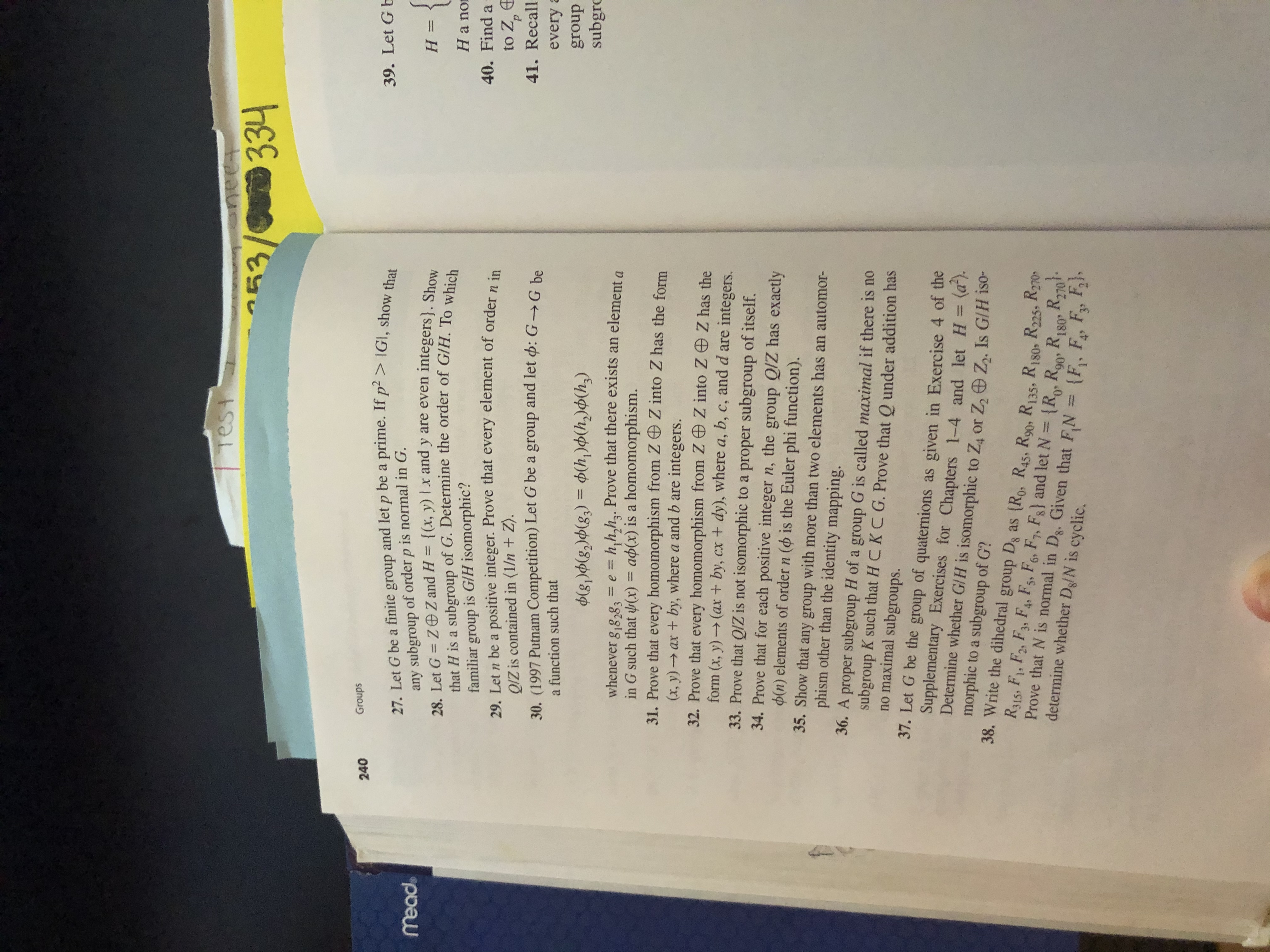help_outlineImage TranscriptioncloseTట ee 5३/७ 334 Groups 240 39. Let G b 27. Let G be a finite group and let p be a prime. If p2> IGI, show that any subgroup of order p is normal in G. 28. Let G ZeZ and H = {(x, y) |x and y are even that H is a subgroup of G. Determine the order of G/H. To which mead integers). Show Н- Нa no familiar group is GIH isomorphic? 29. Let n be a positive integer. Prove that every element of order n in Q/Z is contained in (1/n + Z). 30. (1997 Putnam Competition) Let G be a group and let d: G-G be 40. Find a to Z € р 41. Recall every a function such that group s)(8)8) d(h,)(h,)(h,) subgr whenever g18,83 = e = h,h,hg. Prove that there exists an element a in G such that (x) = ap(x) is a homomorphism. 31. Prove that every homomorphism from Z Z into Z has the form (x, y)- ax + by, where a and b are integers. 32. Prove that every homomorphism from Z Z into Z Z has the form (x, y)-(ax + by, cx + dy), where a, b, c, and d are integers. 33. Prove that Q/Z is not isomorphic to a proper subgroup of itself. 34. Prove that for each positive integer n, the group Q/Z has exactly (n) elements of order n (d is the Euler phi function). 35. Show that any group with more than two elements has an automor- phism other than the identity mapping. 36. A proper subgroup H of a group G is called maximal if there is no subgroup no maximal subgroups. 37. Let G be the group of quaternions as given in Exercise 4 of te Supplementary Exercises for Chapters 1-4 and let H = (a). Determine whether G/H is isomorphic to Za or Z, Z. Is G/H iso- morphic to a subgroup of G? 38. Write the dihedral group Dg as (Ro, R45, Ro0, R135, R180, Rs, R0 R315, F, F2, F, F4, Fs, F6 F, Fs) and let N (Rp, R RI0 R7 Prove that N is normal in Ds. Given that F,N determine whether DglN is cyclic. K such that H CKC G. Prove that O under addition has 90* (F. F F F) 4* fullscreen
check_circle

Step 1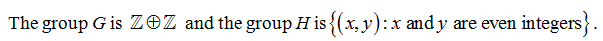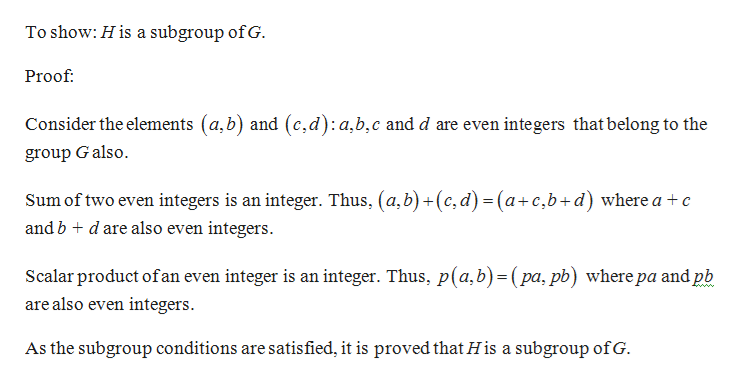help_outlineImage TranscriptioncloseTo show: H is a subgroup of G. Proof Consider the elements (a,b) and (c, d) : a,b,c and d are even integers that belong to the group Galso Sum of two even integers is an integer. Thus, (a,b)(c, d) (a+c,b+d) where a + c and b d are also even integers Scalar product ofan even integer is an integer. Thus, p(a,b)(pa, pb) where pa and pb are also even integers w. As the subgroup conditions are satisfied, it is proved that H is a subgroup ofG. fullscreen
Step 2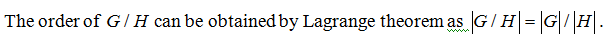...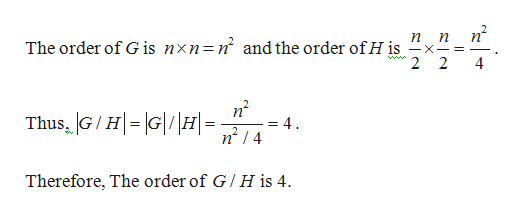help_outlineImage Transcriptionclosen n X The order of G is nxn=n and the order of H is www. 2 4 Thus, G/HG|H=. = 4 Therefore, The order of G/ H is 4 fullscreen

### Want to see the full answer?

See Solution

#### Want to see this answer and more?

Solutions are written by subject experts who are available 24/7. Questions are typically answered within 1 hour.*

See Solution
*Response times may vary by subject and question.
Tagged in

### Math